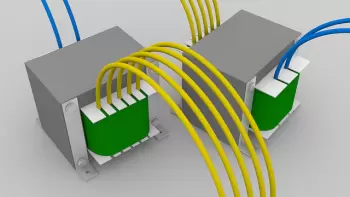# Types of Electrical Circuits, Description and CharacteristicsElectric circuits can be classified in different ways depending on the type of current that circulates in it and the configuration of the connections of the different elements.

## Types of Electrical Circuits Depending on the Current Flow

A possible classification of electrical circuits depends on the type of electrical current they use. Electric current can be of two types: alternating or continuous. Depending on the current used, the circuit elements have to be adapted.

A current inverter is an element that transforms electrical energy from alternating current to direct current or vice versa. For example, the electrical network supplies electricity in alternating current while photovoltaic panels provide direct current.

### Direct Current Circuit

The electric current stays in the same direction all the time.

Although the term direct current is often used to denote a constant voltage, a direct current is not necessarily a constant voltage. The variable voltage current is correct as long as the polarity does not change.

### Alternating Current Circuit

Electric current has both directions; polarity is variable.

Although the term alternating current is often used to denote a sinusoidal alternating current in a power supply, all currents that change direction are alternating, such as triangle waves and some electrical signals.

A sinusoidal alternating current can be single-phase or polyphase.

A polyphase system is a system for generating, distributing and consuming electrical energy that has two or more lines with equal voltages and constant phase differences.

The most widely used polyphase system is the three-phase system.

### Types of Electrical Circuits Depending on the Configuration of Connections

Circuits can be classified depending on how their elements are connected. In this sense, the connections can be in series, in parallel or mixed.

The choice of one type or another of connection will affect the values ​​of voltage, current intensity and resistance according to Ohm's law.

### Series Electrical Circuit

In a series circuit, the terminals and terminals of the devices are configured sequentially and one after the other. That is, the output terminal of one device is connected to the input terminal of the next device.

• The total voltage is equal to the sum of the voltages of all the elements of the circuit. (Vt = V1 + V2).

• The intensity is the same in all branches of the circuit. (I1 = I2 = I3)

### Parallel Electrical Circuit

In a parallel circuit, the input terminals or input terminals of all devices are connected to each other, and the same is the case with the output terminals.

• The potential difference is the same in all branches of the circuit. (V1 = V2 = V3)

• The total intensity is equivalent to the sum of the intensities of each of the branches of the circuit. (It = I1 + I2).

### What Is a Mixed Circuit?

A mixed electronic circuit is a circuit with a series of elements connected in series and others in parallel.

## What Does It Mean That a Circuit Is Open or Closed?

For an electrical circuit to work it is necessary that the current intensity is not interrupted. For electrical energy to flow, the presence of a continuous conductive path between the negative and positive poles is necessary.

In a closed circuit, continuity is guaranteed and the elements of the circuit will perform their function. On the other hand, if there is a cut in the circuit, or simply an open switch, we will say that it is an open circuit and the electrical charges will not be able to flow.

## What Are the Types of Connections in Three-phase Systems?

In three-phase systems, there are two main ways to connect three-phase alternators or loads :

• Triangular connection: The phases are connected from the beginning of each phase to the end of the next.

• Star connection: one side of the generator winding or load is connected to a line and the other side to a common neutral point called neutral and generally connected to ground.

Author:

Published: September 10, 2021
Last review: September 10, 2021